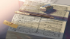Discussion about math, puzzles, games and fun.   Useful symbols: ÷ × ½ √ ∞ ≠ ≤ ≥ ≈ ⇒ ± ∈ Δ θ ∴ ∑ ∫  π  -¹ ² ³ °

You are not logged in.

## #1 2019-08-23 09:41:27

Hannibal lecter
MemberRegistered: 2016-02-11
Posts: 279

### Percentage Calculating

when we add 1000.00 to 5% = 10500.00
and when we multiply 1000.00 * 0.05 = 10500.00

can someone explain how is that happen? I'm weak in algebra so I want to understand this scientifically not just add numbers and multiply
because I know how to multiply and add numbers but I don't know the explanation of that  and the scientific reasons

please explain that for me because I'm about to be a lecturer in the future I want to ask all the questions that giving to me